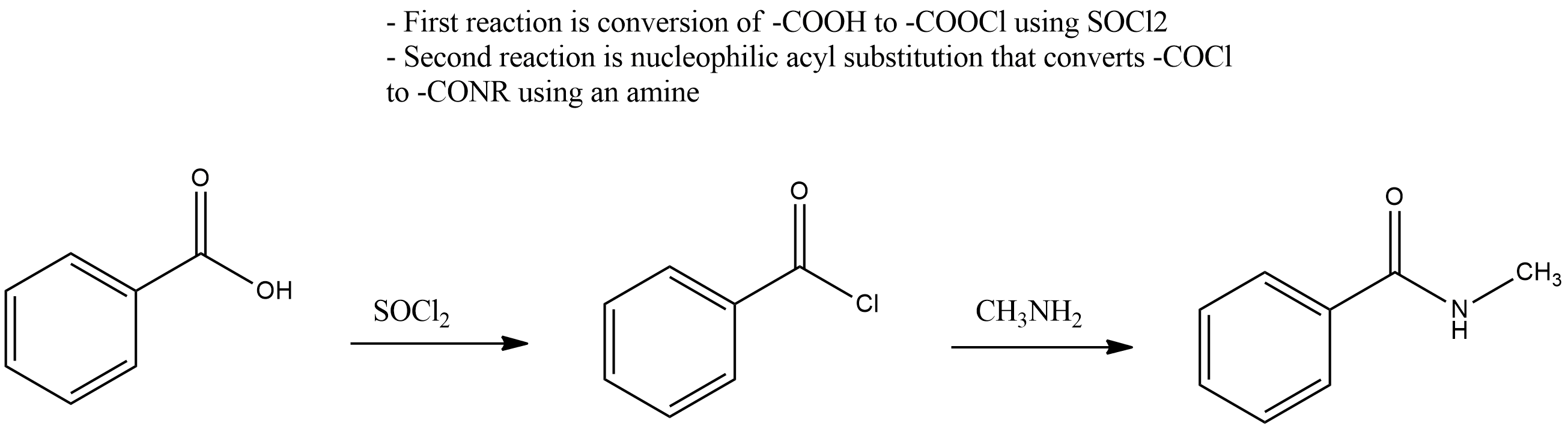# Problem: Consider the structural formula of acetaldehyde below. (a) Give a chemical equation for the reaction between hydroxide ion (HO -) and acetaldehyde in which HO- acts as a Lewis base and acetaldehyde acts as a Lewis acid. IMPORTANT: The Lewis base that you give cannot be a Bronsted-Lowry base; the Lewis acid that you give cannot be a Bronsted-Lowry acid. Note: Use Lewis structures for each reactant/product and use curved arrows appropriately.            (b) Using Lewis structures for each reactant and each product and using curved arrows appropriately to show the flow of electrons in the reactants, give a chemical equation for the reaction between hydroxide ion (HO -) and acetaldehyde in which HO- acts as a Bronsted-Lowry base and acetaldehyde acts as a Bronsted-Lowry acid. Do not give the same answer that you gave in the first part of this question.

83% (74 ratings)
###### Problem Details

Consider the structural formula of acetaldehyde below.

(a) Give a chemical equation for the reaction between hydroxide ion (HO -) and acetaldehyde in which HO- acts as a Lewis base and acetaldehyde acts as a Lewis acid. IMPORTANT: The Lewis base that you give cannot be a Bronsted-Lowry base; the Lewis acid that you give cannot be a Bronsted-Lowry acid. Note: Use Lewis structures for each reactant/product and use curved arrows appropriately.

(b) Using Lewis structures for each reactant and each product and using curved arrows appropriately to show the flow of electrons in the reactants, give a chemical equation for the reaction between hydroxide ion (HO -) and acetaldehyde in which HO- acts as a Bronsted-Lowry base and acetaldehyde acts as a Bronsted-Lowry acid. Do not give the same answer that you gave in the first part of this question.